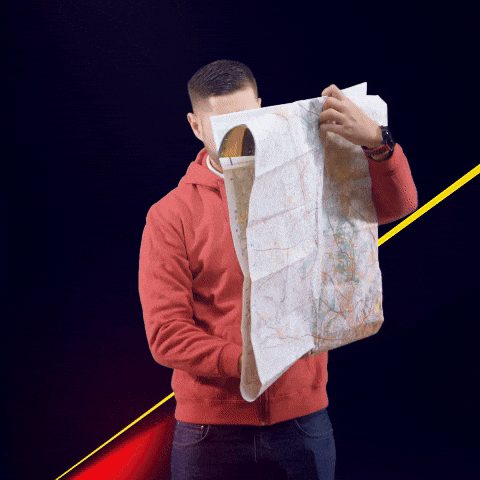# Python Tutorial of OSRM(Open Sourced Routing Machine) and Applications

I came across a wonderful open source project recently — Project OSRM (link) — A modern C++ routing engine for shortest paths in road networks. You can imagine it as a free version of Google Maps API, without live traffic of course. It is very valuable for my work because my current company has large shipping and logistic services. Being able to calculate the distance and directions between locations in a timely fashion will enable us to research and modeling on route optimization, leads generation, etc.

The solution itself is quite straightforward and I am able to setup an API sandbox running in a couple of hours.

First you need to get the OSRM back-end running as a container on your machine. The process is very easy to follow on the project’s github page here. After that you can easily interact with it in python, let’s take a look:

## Package Needed

We need `folium` package to draw the routes on the map and `polyline` to decode the routes from the API output. We will talk about that more later.

``````import requests
import folium
import polyline
``````

## Single Request

You can request the driving route by supply the latitude and longitude of your start and end points, separate by , and ;

``````url = "http://10.22.168.65:9080/route/v1/driving/-117.851364,33.698206;-117.838925,33.672260"
r = requests.get(url)
res = r.json()
res
``````
``````{'code': 'Ok',
'routes': [{'geometry': 'gttlEfyhnUpBtC|k@e`Aro@zo@tf@jXhFaMxSe]lDgKlAqIGwTcIyB',
'legs': [{'steps': [],
'distance': 4995.3,
'duration': 409.1,
'summary': '',
'weight': 422.5}],
'distance': 4995.3,
'duration': 409.1,
'weight_name': 'routability',
'weight': 422.5}],
'waypoints': [{'hint': 'O14sgD1eLIAMAQAASQEAAAAAAAAAAAAAWs1fQprEiEIAAAAAAAAAAIYAAAClAAAAAAAAAAAAAACQBgAAnbv5-EQxAgIcu_n4njECAgAAfwLrq6bJ',
'distance': 15.580755,
'name': '',
'location': [-117.851235, 33.698116]},
'distance': 31.847501,
'name': 'Carlson Avenue',
'location': [-117.838617, 33.672133]}]}
``````

The output is easy to follow. This trip has a distance of 4995 meters and travel time of 409 seconds, with the routes encoded using google’s Polyline Algorithm. We can use python package `polyline` to decode it into coordinates:

``````polyline.decode('gttlEfyhnUpBtC|k@e`Aro@zo@tf@jXhFaMxSe]lDgKlAqIGwTcIyB')
``````
``````[(33.69812, -117.85124),
(33.69755, -117.85199),
(33.69036, -117.84156),
(33.68258, -117.84938),
(33.67623, -117.85344),
(33.67506, -117.85119),
(33.67173, -117.84636),
(33.67086, -117.8444),
(33.67047, -117.84271),
(33.67051, -117.83923),
(33.67213, -117.83862)]
``````

Now this is something we can work with! Lets wrap it into a function:

``````def get_route(pickup_lon, pickup_lat, dropoff_lon, dropoff_lat):

loc = "{},{};{},{}".format(pickup_lon, pickup_lat, dropoff_lon, dropoff_lat)
url = "http://10.22.168.65:9080/route/v1/driving/"
r = requests.get(url + loc)
if r.status_code!= 200:
return {}

res = r.json()
routes = polyline.decode(res['routes']['geometry'])
start_point = [res['waypoints']['location'], res['waypoints']['location']]
end_point = [res['waypoints']['location'], res['waypoints']['location']]
distance = res['routes']['distance']

out = {'route':routes,
'start_point':start_point,
'end_point':end_point,
'distance':distance
}

return out
``````
``````pickup_lon, pickup_lat, dropoff_lon, dropoff_lat = -117.851364,33.698206,-117.838925,33.672260
test_route = get_route(pickup_lon, pickup_lat, dropoff_lon, dropoff_lat)
test_route
``````
``````{'route': [(33.69812, -117.85124),
(33.69755, -117.85199),
(33.69036, -117.84156),
(33.68258, -117.84938),
(33.67623, -117.85344),
(33.67506, -117.85119),
(33.67173, -117.84636),
(33.67086, -117.8444),
(33.67047, -117.84271),
(33.67051, -117.83923),
(33.67213, -117.83862)],
'start_point': [33.698116, -117.851235],
'end_point': [33.672133, -117.838617],
'distance': 4995.3}
``````

## Draw the route on map

Now we have the output nicely organized in coordinates format, let’s use `folium` package to chart the routes and see if it makes sense or not.

``````def get_map(route):

m = folium.Map(location=[(route['start_point'] + route['end_point'])/2,
(route['start_point'] + route['end_point'])/2],
zoom_start=13)

folium.PolyLine(
route['route'],
weight=8,
color='blue',
opacity=0.6

folium.Marker(
location=route['start_point'],
icon=folium.Icon(icon='play', color='green')

folium.Marker(
location=route['end_point'],
icon=folium.Icon(icon='stop', color='red')

return m
``````
``````get_map(test_route)
``````

I just randomly pick two points in Irvine, CA and the route looks pretty good!

## Benchmarking

If I want to use this API to processing data for me, I would like to know how fast it can handle my requests. Here I randomly generated another 1000 coordinates and request the routes from our docker backend as a mini stress test:

``````import numpy as np
import pandas as pd
lon1 = np.random.uniform(-117.4,-118, 1000).round(6)
lon2 = np.random.uniform(-117.4,-118, 1000).round(6)
lat1 = np.random.uniform(33.6,33.8, 1000).round(6)
lat2 = np.random.uniform(33.6,33.8, 1000).round(6)
df = pd.DataFrame({'pickup_lon': lon1,
'pickup_lat': lat1,
'dropoff_lon': lon2,
'dropoff_lat': lat2,
})
``````
``````df.head()
``````
pickup_lon pickup_lat dropoff_lon dropoff_lat
0 -117.650723 33.696095 -117.400615 33.675578
1 -117.653614 33.713656 -117.920080 33.679549
2 -117.484076 33.671013 -117.960667 33.741495
3 -117.599436 33.656727 -117.481877 33.643613
4 -117.968429 33.776134 -117.469914 33.739116
``````%%time
df['routes'] = df.apply(lambda x: get_route(x['pickup_lon'],
x['pickup_lat'],
x['dropoff_lon'],
x['dropoff_lat']), axis=1)
``````
``````CPU times: user 1.55 s, sys: 118 ms, total: 1.67 s
Wall time: 5.72 s
``````

Not bad at all! With single container and it can finish the request async in 6 seconds. If we put it on a multiple node docker swarm cluster with proper load balancer, I believe the performance will be very staisfactory.

Check with a random data I requested:

``````get_map(df.loc[900,'routes'])
``````

## Potential applications

Now we have seen the beauty of the OSRM. You can imagine how many use cases it could potentially has. I actually used it to generate features in one Kaggle competition — NYC taxi fare prediction (link). In this competition, you were asked to predict the taxi fares given some basic features including the pickup and dropoff coordinates. As we all know that Haversine distance is different than the actually driving distance, especially in NYC. My intuition is that using the predicted driving distance will increase the model accuracy. Because that is how the taxi fares are calculated anyway. I was absolutely right. By adding this trip distance to the data, I am able to achieve the score of 3.09 which is about 3001500 on the leaderboard, using just 10% of the data! (full dataset is too big to work with on my laptop) I will publish my detailed approach in the next posts if you are interested.

So what are you waiting for? Starting routing now!##### Michael Yan
###### Staff Data Scientist @ SpotHero

A student of the last forbidden art of Data Science.